# How to image a virus?

Christian Zuniga, PhD

Viruses are very, very small. Although their existence was suspected since the 19th century, they were too small to be seen . Viruses are so small that taking a picture with an ordinary light microscope would not detect them. Figure 1 shows an image of a MERS Coronavirus taken with an electron microscope . The diameter of the virus is on the order of 100 nm (or 0.0000001 m). For comparison, the width of a human hair is around 80 um so a virus is around 8000 times smaller.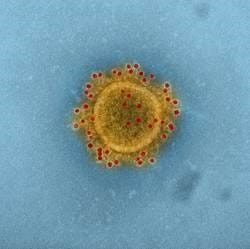Figure 1 EM Image of a virus

Why is it difficult for ordinary light to image a virus? To simplify the situation lets model the virus as an opaque thin disk of diameter d as shown in Figure 2. When illuminated from behind, the virus should completely block the light and produce a shadow in front as the right side of Figure 2 shows.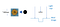Figure 2 Virus modeled as a thin opaque disk (left). Ideal image of illuminated virus as seen from a side.

The ideal image in Figure 2 assumes the light travels in straight lines as particles. However light travels as a wave and disperses as it hits the disk. The effect is similar to a water wave hitting an obstacle as shown in Figure 3. The amount of dispersion depends on the relative size of the disk compared to the wave.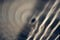Figure 3 Water wave dispersing as it hits an obstacle 

Wave propagation is described by a partial differential equation which is linear. This implies any wave can be described as a superposition or linear combination of elementary waves, each being a solution of the wave equation. The decomposition of light into simpler components is vividly illustrated by a prism as shown in Figure 4.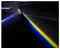Figure 4 Prism decomposing white light into elementary colors 

One set of such elementary waves are called plane waves as shown in Figure 4. These are waves whose surfaces of constant phase such as a peak lie on a plane. These elementary waves are described by a wave vector k=[kx, ky, kz] giving its direction of propagation and take the form below where ‘A’ is the amplitude. There is also a sinusoidal time component but is not important for this discussion. The waves have a spatial period called the wavelength (symbolized by λ ) and is inversely related to the wavenumber by k = 2 π/ λ.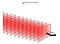Figure 5 Representation of a plane wave. The planes represent surfaces of constant phase.

The image of a virus can equally be represented as a superposition of plane waves, each traveling in a different direction and having different amplitudes . Figure 6 shows this concept for 2 dimensions, x and z. If the image is called v(x) then it is expressed as a superposition of plane waves as shown below. The origin is at z=0 so the z component is equal to 1 and doesn’t appear.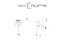Figure 6 Expansion of image into a sum of plane waves traveling in different directions

The amplitude of each wave V(kx) is given by the Fourier Transform of the wave image v(x). As the expression shows, an infinite number of waves are needed to recreate the virus image v(x). However physical constraints impose a limit on the number of waves present. As the figure shows the direction of travel can also be expressed by the angle the wave makes with the z axis. The wavenumber can then be expressed as k = [k sin(a) , k cos(a) ]. Since the magnitude of the sine is bounded by 1, the x component of the wavenumber can’t exceed 2π / λ.

The spatial frequency fx must have a magnitude less than or equal the inverse of the wavelength. Higher frequencies can’t be used in reconstructing the image. The effect of this filtering is to blur the image. Figure 7 shows the amplitude spectrum and the reconstruction with a finite number of waves if the wavelength was much smaller than the size of the virus, the reconstruction will resemble the ideal shape although not perfectly because the wavelength never goes to 0 (bandwidth is limited). Visible light has a range of ~380–740 nm. Figure 8 shows the reconstruction when the waves are limited by the lower value of 1/380 nm. Some signal is still present. A microscope would add additional filtering lowering the cut-off frequency to NA/λ (in free space) but would not completely eliminate the signal. However this was an isolated virus. There were no other particles around that would cause interference. Lenses have aberrations. The virus was assumed opaque which is not the case and ever-present noise would degrade the signal.

Nevertheless, it remains true that to improve spatial resolution, lower wavelengths should be used. Although there are electromagnetic wavelengths in the nm range (x-rays) there use is complicated by other factors. Electrons can also provide shorter wavelengths and have been successfully used to image the nano world.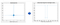Figure 7 Reconstruction of image when wavelength is much smaller than diameter of virus ( delta function not shown for clarity)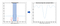Figure 6 Image reconstruction with spectrum bandwidth limited to 1/380nm from visible light.

References

 Goodman “Introduction to Fourier Optics” 4th Edition, McMillan Learning 2017

Christian Zuniga has worked as a lecturer in San Jose State University in data mining. He worked in OPC modeling and is interested in machine learning.

## More from Christian Zuniga

Christian Zuniga has worked as a lecturer in San Jose State University in data mining. He worked in OPC modeling and is interested in machine learning.